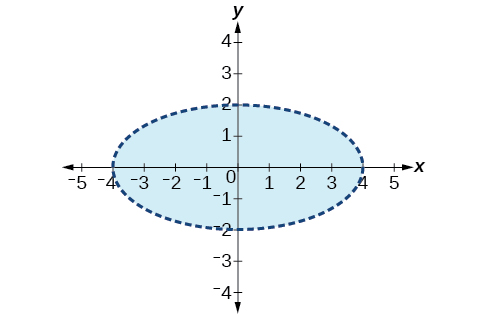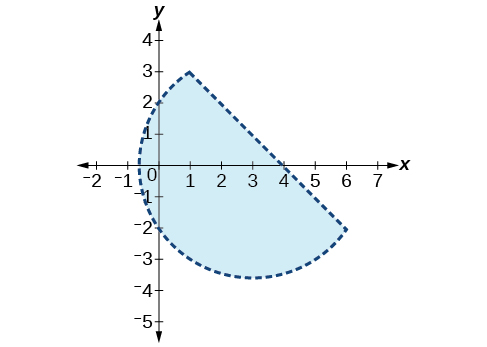# 9.8 Solving systems with cramer's rule  (Page 8/11)

 Page 8 / 11

$\left(10,-10,10\right)$

$\begin{array}{r}\hfill 5x+3y-z=5\text{\hspace{0.17em}}\text{\hspace{0.17em}}\text{\hspace{0.17em}}\\ \hfill 3x-2y+4z=13\\ \hfill 4x+3y+5z=22\end{array}$

$\begin{array}{r}x+y+z=1\\ 2x+2y+2z=1\\ 3x+3y=2\end{array}$

No solutions exist.

$\begin{array}{l}\text{\hspace{0.17em}}\text{\hspace{0.17em}}3x+2y-z=-10\hfill \\ \text{\hspace{0.17em}}\text{\hspace{0.17em}}\text{\hspace{0.17em}}\text{\hspace{0.17em}}x-y+2z=7\hfill \\ -x+3y+z=-2\hfill \end{array}$

$\left(-1,-2,3\right)$

$\begin{array}{r}\hfill 3x+4z=-11\\ \hfill x-2y=5\text{\hspace{0.17em}}\text{\hspace{0.17em}}\text{\hspace{0.17em}}\text{\hspace{0.17em}}\text{\hspace{0.17em}}\text{\hspace{0.17em}}\text{\hspace{0.17em}}\\ \hfill 4y-z=-10\end{array}$

$\begin{array}{r}2x-3y+z=0\\ 2x+4y-3z=0\\ 6x-2y-z=0\end{array}$

$\left(x,\frac{8x}{5},\frac{14x}{5}\right)$

$\begin{array}{r}6x-4y-2z=2\\ 3x+2y-5z=4\\ 6y-7z=5\end{array}$

For the following exercises, write a system of equations to solve each problem. Solve the system of equations.

Three odd numbers sum up to 61. The smaller is one-third the larger and the middle number is 16 less than the larger. What are the three numbers?

11, 17, 33

A local theatre sells out for their show. They sell all 500 tickets for a total purse of $8,070.00. The tickets were priced at$15 for students, $12 for children, and$18 for adults. If the band sold three times as many adult tickets as children’s tickets, how many of each type was sold?

## Systems of Nonlinear Equations and Inequalities: Two Variables

For the following exercises, solve the system of nonlinear equations.

$\begin{array}{l}\begin{array}{l}\\ y={x}^{2}-7\end{array}\hfill \\ y=5x-13\hfill \end{array}$

$\left(2,-3\right),\left(3,2\right)$

$\begin{array}{l}\begin{array}{l}\\ y={x}^{2}-4\end{array}\hfill \\ y=5x+10\hfill \end{array}$

$\begin{array}{l}{x}^{2}+{y}^{2}=16\hfill \\ \text{\hspace{0.17em}}\text{\hspace{0.17em}}\text{\hspace{0.17em}}\text{\hspace{0.17em}}\text{\hspace{0.17em}}\text{\hspace{0.17em}}\text{\hspace{0.17em}}\text{\hspace{0.17em}}\text{\hspace{0.17em}}\text{\hspace{0.17em}}\text{\hspace{0.17em}}\text{\hspace{0.17em}}\text{\hspace{0.17em}}\text{\hspace{0.17em}}\text{\hspace{0.17em}}\text{\hspace{0.17em}}y=x-8\hfill \end{array}$

No solution

$\begin{array}{l}{x}^{2}+{y}^{2}=25\hfill \\ \text{\hspace{0.17em}}\text{\hspace{0.17em}}\text{\hspace{0.17em}}\text{\hspace{0.17em}}\text{\hspace{0.17em}}\text{\hspace{0.17em}}\text{\hspace{0.17em}}\text{\hspace{0.17em}}\text{\hspace{0.17em}}\text{\hspace{0.17em}}\text{\hspace{0.17em}}\text{\hspace{0.17em}}\text{\hspace{0.17em}}\text{\hspace{0.17em}}\text{\hspace{0.17em}}\text{\hspace{0.17em}}y={x}^{2}+5\hfill \end{array}$

$\begin{array}{r}{x}^{2}+{y}^{2}=4\\ y-{x}^{2}=3\end{array}$

No solution

For the following exercises, graph the inequality.

$y>{x}^{2}-1$

$\frac{1}{4}{x}^{2}+{y}^{2}<4$For the following exercises, graph the system of inequalities.

$\begin{array}{l}{x}^{2}+{y}^{2}+2x<3\hfill \\ \text{\hspace{0.17em}}\text{\hspace{0.17em}}\text{\hspace{0.17em}}\text{\hspace{0.17em}}\text{\hspace{0.17em}}\text{\hspace{0.17em}}\text{\hspace{0.17em}}\text{\hspace{0.17em}}\text{\hspace{0.17em}}\text{\hspace{0.17em}}\text{\hspace{0.17em}}\text{\hspace{0.17em}}\text{\hspace{0.17em}}\text{\hspace{0.17em}}\text{\hspace{0.17em}}\text{\hspace{0.17em}}\text{\hspace{0.17em}}\text{\hspace{0.17em}}\text{\hspace{0.17em}}\text{\hspace{0.17em}}\text{\hspace{0.17em}}\text{\hspace{0.17em}}\text{\hspace{0.17em}}\text{\hspace{0.17em}}\text{\hspace{0.17em}}\text{\hspace{0.17em}}\text{\hspace{0.17em}}\text{\hspace{0.17em}}\text{\hspace{0.17em}}y>-{x}^{2}-3\hfill \end{array}$

$\begin{array}{l}{x}^{2}-2x+{y}^{2}-4x<4\hfill \\ \text{\hspace{0.17em}}\text{\hspace{0.17em}}\text{\hspace{0.17em}}\text{\hspace{0.17em}}\text{\hspace{0.17em}}\text{\hspace{0.17em}}\text{\hspace{0.17em}}\text{\hspace{0.17em}}\text{\hspace{0.17em}}\text{\hspace{0.17em}}\text{\hspace{0.17em}}\text{\hspace{0.17em}}\text{\hspace{0.17em}}\text{\hspace{0.17em}}\text{\hspace{0.17em}}\text{\hspace{0.17em}}\text{\hspace{0.17em}}\text{\hspace{0.17em}}\text{\hspace{0.17em}}\text{\hspace{0.17em}}\text{\hspace{0.17em}}\text{\hspace{0.17em}}\text{\hspace{0.17em}}\text{\hspace{0.17em}}\text{\hspace{0.17em}}\text{\hspace{0.17em}}\text{\hspace{0.17em}}\text{\hspace{0.17em}}\text{\hspace{0.17em}}\text{\hspace{0.17em}}\text{\hspace{0.17em}}\text{\hspace{0.17em}}\text{\hspace{0.17em}}\text{\hspace{0.17em}}\text{\hspace{0.17em}}\text{\hspace{0.17em}}\text{\hspace{0.17em}}\text{\hspace{0.17em}}\text{\hspace{0.17em}}\text{\hspace{0.17em}}\text{\hspace{0.17em}}\text{\hspace{0.17em}}y<-x+4\hfill \end{array}$$\begin{array}{l}{x}^{2}+{y}^{2}<1\hfill \\ \text{\hspace{0.17em}}\text{\hspace{0.17em}}\text{\hspace{0.17em}}\text{\hspace{0.17em}}\text{\hspace{0.17em}}\text{\hspace{0.17em}}\text{\hspace{0.17em}}\text{\hspace{0.17em}}\text{\hspace{0.17em}}\text{\hspace{0.17em}}\text{\hspace{0.17em}}\text{\hspace{0.17em}}\text{\hspace{0.17em}}{y}^{2}

## Partial Fractions

For the following exercises, decompose into partial fractions.

$\frac{-2x+6}{{x}^{2}+3x+2}$

$\frac{2}{x+2},\frac{-4}{x+1}$

$\frac{10x+2}{4{x}^{2}+4x+1}$

$\frac{7x+20}{{x}^{2}+10x+25}$

$\frac{7}{x+5},\frac{-15}{{\left(x+5\right)}^{2}}$

$\frac{x-18}{{x}^{2}-12x+36}$

$\frac{-{x}^{2}+36x+70}{{x}^{3}-125}$

$\frac{3}{x-5},\frac{-4x+1}{{x}^{2}+5x+25}$

$\frac{-5{x}^{2}+6x-2}{{x}^{3}+27}$

$\frac{{x}^{3}-4{x}^{2}+3x+11}{{\left({x}^{2}-2\right)}^{2}}$

$\frac{x-4}{\left({x}^{2}-2\right)},\frac{5x+3}{{\left({x}^{2}-2\right)}^{2}}$

$\frac{4{x}^{4}-2{x}^{3}+22{x}^{2}-6x+48}{x{\left({x}^{2}+4\right)}^{2}}$

## Matrices and Matrix Operations

For the following exercises, perform the requested operations on the given matrices.

$A=\left[\begin{array}{rr}\hfill 4& \hfill -2\\ \hfill 1& \hfill 3\end{array}\right],B=\left[\begin{array}{rrr}\hfill 6& \hfill 7& \hfill -3\\ \hfill 11& \hfill -2& \hfill 4\end{array}\right],C=\left[\begin{array}{r}\hfill \begin{array}{cc}6& 7\\ 11& -2\end{array}\\ \hfill \begin{array}{cc}14& 0\end{array}\end{array}\right],D=\left[\begin{array}{rrr}\hfill 1& \hfill -4& \hfill 9\\ \hfill 10& \hfill 5& \hfill -7\\ \hfill 2& \hfill 8& \hfill 5\end{array}\right],E=\left[\begin{array}{rrr}\hfill 7& \hfill -14& \hfill 3\\ \hfill 2& \hfill -1& \hfill 3\\ \hfill 0& \hfill 1& \hfill 9\end{array}\right]$

$-4A$

$\left[\begin{array}{cc}-16& 8\\ -4& -12\end{array}\right]$

$10D-6E$

$B+C$

undefined; dimensions do not match

$AB$

$BA$

undefined; inner dimensions do not match

$BC$

$CB$

$\left[\begin{array}{ccc}113& 28& 10\\ 44& 81& -41\\ 84& 98& -42\end{array}\right]$

$DE$

$ED$

$\left[\begin{array}{ccc}-127& -74& 176\\ -2& 11& 40\\ 28& 77& 38\end{array}\right]$

$EC$

$CE$

undefined; inner dimensions do not match

${A}^{3}$

## Solving Systems with Gaussian Elimination

For the following exercises, write the system of linear equations from the augmented matrix. Indicate whether there will be a unique solution.

$\begin{array}{l}x-3z=7\\ y+2z=-5\text{\hspace{0.17em}}\end{array}$ with infinite solutions

For the following exercises, write the augmented matrix from the system of linear equations.

$\begin{array}{l}\\ \begin{array}{r}\hfill -2x+2y+z=7\\ \hfill 2x-8y+5z=0\\ \hfill 19x-10y+22z=3\end{array}\end{array}$

$\begin{array}{l}\text{\hspace{0.17em}}\text{\hspace{0.17em}}\text{\hspace{0.17em}}\text{\hspace{0.17em}}\text{\hspace{0.17em}}4x+2y-3z=14\hfill \\ -12x+3y+z=100\hfill \\ \text{\hspace{0.17em}}\text{\hspace{0.17em}}\text{\hspace{0.17em}}\text{\hspace{0.17em}}\text{\hspace{0.17em}}9x-6y+2z=31\hfill \end{array}$

$\begin{array}{r}\hfill x+3z=12\text{\hspace{0.17em}}\\ \hfill -x+4y=0\text{\hspace{0.17em}}\text{\hspace{0.17em}}\text{\hspace{0.17em}}\text{\hspace{0.17em}}\\ \hfill y+2z=-7\end{array}$

For the following exercises, solve the system of linear equations using Gaussian elimination.

$\begin{array}{r}3x-4y=-7\\ -6x+8y=14\end{array}$

$\begin{array}{r}3x-4y=1\\ -6x+8y=6\end{array}$

No solutions exist.

$\begin{array}{l}\begin{array}{l}\\ -1.1x-2.3y=6.2\end{array}\hfill \\ -5.2x-4.1y=4.3\hfill \end{array}$

$\begin{array}{r}\hfill 2x+3y+2z=1\text{\hspace{0.17em}}\text{\hspace{0.17em}}\text{\hspace{0.17em}}\text{\hspace{0.17em}}\text{\hspace{0.17em}}\\ \hfill -4x-6y-4z=-2\\ \hfill 10x+15y+10z=0\text{\hspace{0.17em}}\text{\hspace{0.17em}}\text{\hspace{0.17em}}\text{\hspace{0.17em}}\text{\hspace{0.17em}}\end{array}$

No solutions exist.

$\begin{array}{r}\hfill -x+2y-4z=8\text{\hspace{0.17em}}\text{\hspace{0.17em}}\text{\hspace{0.17em}}\text{\hspace{0.17em}}\\ \hfill 3y+8z=-4\\ \hfill -7x+y+2z=1\text{\hspace{0.17em}}\text{\hspace{0.17em}}\text{\hspace{0.17em}}\text{\hspace{0.17em}}\end{array}$

## Solving Systems with Inverses

For the following exercises, find the inverse of the matrix.

$\left[\begin{array}{rr}\hfill -0.2& \hfill 1.4\\ \hfill 1.2& \hfill -0.4\end{array}\right]$

$\frac{1}{8}\left[\begin{array}{cc}2& 7\\ 6& 1\end{array}\right]$

$\left[\begin{array}{rr}\hfill \frac{1}{2}& \hfill -\frac{1}{2}\\ \hfill -\frac{1}{4}& \hfill \frac{3}{4}\end{array}\right]$

$\left[\begin{array}{ccc}12& 9& -6\\ -1& 3& 2\\ -4& -3& 2\end{array}\right]$

No inverse exists.

$\left[\begin{array}{ccc}2& 1& 3\\ 1& 2& 3\\ 3& 2& 1\end{array}\right]$

what is the importance knowing the graph of circular functions?
can get some help basic precalculus
What do you need help with?
Andrew
how to convert general to standard form with not perfect trinomial
can get some help inverse function
ismail
Rectangle coordinate
how to find for x
it depends on the equation
Robert
whats a domain
The domain of a function is the set of all input on which the function is defined. For example all real numbers are the Domain of any Polynomial function.
Spiro
foci (–7,–17) and (–7,17), the absolute value of the differenceof the distances of any point from the foci is 24.
difference between calculus and pre calculus?
give me an example of a problem so that I can practice answering
x³+y³+z³=42
Robert
dont forget the cube in each variable ;)
Robert
of she solves that, well ... then she has a lot of computational force under her command ....
Walter
what is a function?
I want to learn about the law of exponent
explain this
what is functions?
A mathematical relation such that every input has only one out.
Spiro
yes..it is a relationo of orders pairs of sets one or more input that leads to a exactly one output.
Mubita
Is a rule that assigns to each element X in a set A exactly one element, called F(x), in a set B.
RichieRich
If the plane intersects the cone (either above or below) horizontally, what figure will be created?
can you not take the square root of a negative number
No because a negative times a negative is a positive. No matter what you do you can never multiply the same number by itself and end with a negative
lurverkitten
Actually you can. you get what's called an Imaginary number denoted by i which is represented on the complex plane. The reply above would be correct if we were still confined to the "real" number line.
LiamByByBy Yacoub JayoghliBy Sarah WarrenBy OpenStaxBy Stephen VoronBy Janet ForresterBy Yacoub JayoghliBy Rohini AjayBy Marriyam RanaBy Brenna FikeBy Janet Forrester